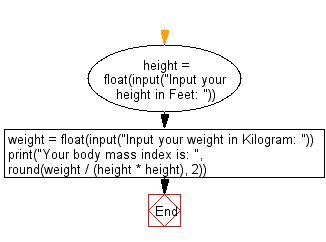﻿ Python: Calculate body mass index - w3resource# Python: Calculate body mass index

## Python Basic: Exercise-66 with Solution

Write a Python program to calculate body mass index.

Pictorial Presentation:Sample Solution:-

Python Code:

``````height = float(input("Input your height in Feet: "))
weight = float(input("Input your weight in Kilogram: "))
print("Your body mass index is: ", round(weight / (height * height), 2))
```
```

Sample Output:

```Input your height in Feet:  6
Input your weight in Kilogram:  65
Your body mass index is:  1.81
```

Flowchart:## Visualize Python code execution:

The following tool visualize what the computer is doing step-by-step as it executes the said program:

Python Code Editor:

Have another way to solve this solution? Contribute your code (and comments) through Disqus.

What is the difficulty level of this exercise?

Test your Programming skills with w3resource's quiz.

﻿

## Python: Tips of the Day

Clamps num within the inclusive range specified by the boundary values x and y:

Example:

```def tips_clamp_num(num,x,y):
return max(min(num, max(x, y)), min(x, y))
print(tips_clamp_num(2, 4, 6))
print(tips_clamp_num(1, -1, -6))
```

Output:

```4
-1
```